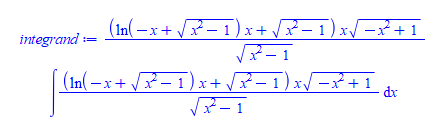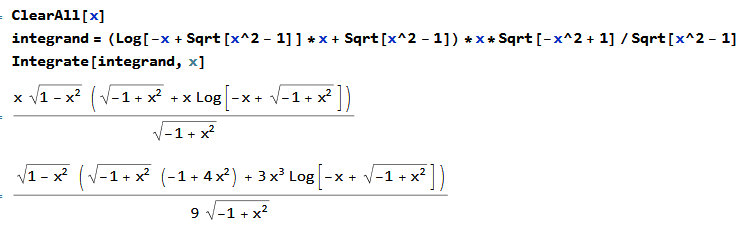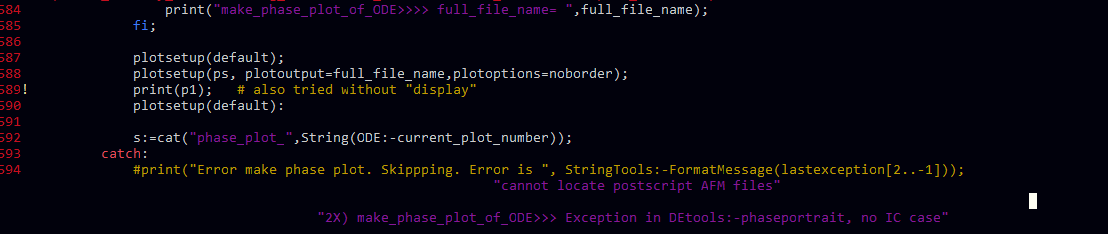## 4 dimensions matrix...

I need create a matrix with 4 dimensions, but I don't know how can I do this. The variable that I need store could be write in this form:

>S[i,j,k,l];

I don't want write this with subscript but like a matrix.

## Re: Substituting an expression (different)...

Hello there,

This is another issue, associated with substitution. In the following Maple expressions, I tried to substitutte the denominator of 'eq_K1_m4' in order to make it as 'eq_K1_m4_desired', but did not get any success (yet).

Therefore, would you have a look at this issue to see if the intended goal can be achieved?

 > restart;
 > with(LinearAlgebra):
 > with(DynamicSystems):
 > interface(imaginaryunit=j):
 > eq_K1_m4 := K__1 = E__q0*(R__T*E__B*sin(delta) + X__Td*E__B*cos(delta))/(L__aqs*L__l + L__aqs*X__E + L__aqs*L__ads_p + L__l^2 + 2*L__l*X__E + L__l*L__ads_p + R__E^2 + 2*R__E*R__a + R__a^2 + X__E^2 + X__E*L__ads_p) + (X__q - X__dp)*i__q0*(X__Tq*E__B*sin(delta) - R__T*E__B*cos(delta))/(L__aqs*L__l + L__aqs*X__E + L__aqs*L__ads_p + L__l^2 + 2*L__l*X__E + L__l*L__ads_p + R__E^2 + 2*R__E*R__a + R__a^2 + X__E^2 + X__E*L__ads_p);(1)
 > eq_K1_m4_desired := K__1 = E__q0*(R__T*E__B*sin(delta) + X__Td*E__B*cos(delta))/Dx + (X__q - X__dp)*i__q0*(X__Tq*E__B*sin(delta) - R__T*E__B*cos(delta))/Dx;(2)
 > eq_Dx := Dx = L__aqs*L__l + L__aqs*X__E + L__aqs*L__ads_p + L__l^2 + 2*L__l*X__E + L__l*L__ads_p + R__E^2 + 2*R__E*R__a + R__a^2 + X__E^2 + X__E*L__ads_p;(3)
 > denom(op(1, rhs(eq_K1_m4))) - rhs(eq_Dx); # checking to see if the denominator expression is the same as the expression of Dx(4)
 > denom(op(2, rhs(eq_K1_m4))) - rhs(eq_Dx); # checking to see if the denominator expression is the same as the expression of Dx(5)
 > # 1
 > map2(applyrule, eq_Dx, eq_K1_m4); # did not work.(6)
 > # 2
 > subs(eq_Dx, eq_K1_m4); # did not work.(7)
 > # 3
 > simplify(eq_K1_m4, {Dx = L__aqs*L__l + L__aqs*X__E + L__aqs*L__ads_p + L__l^2 + 2*L__l*X__E + L__l*L__ads_p + R__E^2 + 2*R__E*R__a + R__a^2 + X__E^2 + X__E*L__ads_p}, [Dx]); # did not work.(8)
 > # 4
 > algsubs(eq_Dx, eq_K1_m4); # did not work.(9)
 > # 5
 > applyrule(L__aqs*L__l + L__aqs*X__E + L__aqs*L__ads_p + L__l^2 + 2*L__l*X__E + L__l*L__ads_p + R__E^2 + 2*R__E*R__a + R__a^2 + X__E^2 + X__E*L__ads_p = Dx, eq_K1_m4); # did not work.(10)
 >

## workaround to integrating this...

I am surprised Maple could not do this integral. Any ideas how to make it do it?

restart;
integrand:=(ln(-x + sqrt(x^2 - 1))*x + sqrt(x^2 - 1))*x*sqrt(-x^2 + 1)/sqrt(x^2 - 1);
int(integrand,x)For reference, another software gives

ClearAll[x]
integrand = (Log[-x + Sqrt[x^2 - 1]]*x + Sqrt[x^2 - 1])*x*Sqrt[-x^2 + 1]/Sqrt[x^2 - 1]
Integrate[integrand, x]Which I verified to be correct.

Any workarounds? I tried assuming x>0 for example. Not sure what else to try now. I am sure with some pre-processing Maple can do it.

Maple 2023

## How to solve this error...

Can you give the maple code for Shooting method/ Kellor Box Method for this problem.

mvp-1.mw

## Maple Conference 2023: Call for Participation

by: Maple , Maple Learn

We are happy to announce another Maple Conference this year, to be held October 26 and 27, 2023!

It will be a free virtual event again this year, and it will be an excellent opportunity to meet other members of the Maple community and get the latest news about our products. More importantly, it's a chance for you to share the work work you've been doing with Maple and Maple Learn. There are two ways to do this.

First, we have just opened the Call for Participation. We are inviting submissions of presentation proposals on a range of topics related to Maple, including Maple in education, algorithms and software, and applications. We also encourage submission of proposals related to Maple Learn.

You can find more information about the themes of the conference and how to submit a presentation proposal at the Call for Participation page. Applications are due July 11, 2023.

Presenters will have the option to submit papers and articles to a special Maple Conference issue of the Maple Transactions journal after the conference.

The second way in which to share your work is through our Maple Art Gallery and Creative Works Showcase. Details on how to submit your work, due September 14, 2023, are given on the Web page.

Registration for attending the conference will open later this month. Watch for further announcements in the coming weeks.

I encourage all of you here in the Maple Primes community to consider joining us for this event, whether as a presenter or attendee!

Paulina Chin
Contributed Program Chair

## Why does Maple put a strange set of variables in m...

I was unsure if this should be a post or a question, or how to word it properly as I feel the attached file is the best way to see my issue.

However, when doing a computation involing the D_ specifically D_[mu](F[~mu,~nu]) where is a tensor, Maple is putting in strange "variables" into the output of my expression. I set the spacetime variables myself to tau,x,y,z and in the output there is combinations which appear as x(tau),y(tau) which has completely baffled me.

Any ideas/help would be greatly appreciated.

EinsteinPert.mw

EDIT: The problem is resolved when the Physics[Vectors] package is removed and replaced by VectorCalculus for the Lapacian. Still curious as why the Physics[Vectors] package causes this issue. (I of course could just brute force the Laplacian but I thought I would use the power of Maple for this).

## How do I label the curves in Maple graph...

How do I label the curves in Maple graph.

Please, I need commands to tweak my maple 18 graph.

## what the error"couldnt store" means and how can f...

Hi. what the error"couldnt store" means and how can fix it? the file has been attached

## How to get the answer of these integrals with Mapl...

Hi,
I want to have the value of the following two integrals numerically for any arbitrary value of kc  and kh. (Physically the answers of the integrals should be real.)
Is it possible to obtain the analytical solution of these integrals with Meple, in terms of parameters kc, kh, deltac, deltah and sigma ?
Thanks

1.mw

## How do I find a unknown values in boundary value p...

I have some ODE system contains unknown variables with  boundary conditions  (see the attachment). How to solve these system and find the vales of unknown variables.

In this problem, the boundary conditions tends to 0, when x tends to infinity.  BVP_with_parameters.mw(1)(2)## How do I plot Basins of Attraction in maple?...

Greetings! I have solved a nonlinear equation by Newton iterative method. Now i want to plot basins of attraction. Being a beginner, I have no clue how to plot it? Its totally different rom other simple plotting commands. Need help in this regard.Thank

## "cannot locate postscript AFM files" Shows only wh...

When running "C:\Program Files\Maple 2023\bin.X86_64_WINDOWS\cmaple.exe" on my application which makes plots and export them to ps, sometimes I get an exception

"cannot locate postscript AFM files"

This happens on some plots. Not all. The strange thing, using the same exact run, but from the worksheet, it works fine and I get the .ps generated with no such error. This only happens when running from the command line. It happens here


try
plotsetup(ps, plotoutput="plot.ps",plotoptions=noborder);
print(p1);
plotsetup(default):
catch:
print(StringTools:-FormatMessage( lastexception[2..-1] ));
end try;



where p1 above is a plot that was generated earlier OK with no error. I made sure there is no font settings on the plot or any extra options that might cause a problem.

I am not able to make MWE as this problem only shows deep inside my application and only when calling Maple from command line and only shows up on very few plots for some reason I do not understand.  When I made a small .mpl with same code used to make the plot, and run it from command line, the error do not show up. It only shows up when running the whole application from the command line.

My question is: How does Maple find these postscript AFM files? It looks like the postscript driver used by command like Maple is older than the one used by the frontend/GUI and this is why this error only shows from the command line. Has anyone seen such a Maple error before?

I have no idea where to find these AFM postscript files it says it can't find and what to do now. I am on windows 10.

Any suggestion what to lookfor and anything else I can do to help me find the cause of this?

If it is any help, here is the MWE I tried to reproduce this with. But this generated no error. Call this foo.mpl

foo:=proc()
local p1,ode,y,x;
ode:=3*y(x)+x*diff(y(x),x) = 2*x^5;
p1 := DEtools:-phaseportrait(ode,y(x),x=-1..5,[y(2)=1],y=-1000..1000):
try
plotsetup(ps, plotoutput=cat("C:/tmp/plot.ps"),plotoptions=noborder);
print(p1);   # also tried without "display"
plotsetup(default):
catch:
print(StringTools:-FormatMessage( lastexception[2..-1] ));
print("Exception");
end try;
end proc;

foo();

Then run

C:\tmp>"C:\Program Files\Maple 2023\bin.X86_64_WINDOWS\cmaple.exe"  foo.mpl

No error. The error only shows in my large application.

"cannot locate postscript AFM files"

Will keep trying to make a MWE, but for 3 hrs now, no luck yet.

Update

if lasterrorlocus<>'lasterrorlocus' then
print(lasterrorlocus);
showsource();
end if; 

Inside the catch, which print the exact location of the exception generated. It is at print(p1) as you seeagain, same code runs with no problem from the worksheet. This only shows when running from command line. Will keep trying to make MWE...

## keeping special math function names as is when tra...

When translating Maple expression with special math functions, Maple does not use the same familar name shown in worksheet, but replaces these special functions with one letter.

This makes it very hard when looking at the equations in latex and the pdf to know what these special functions are.

Is there an option to keep the same name used in Maple but use the \operatorname{name} in the latex generated? This will go a long way to improve the output and make one understand what they are looking at.

Here are examples below. Notice as side note, that Maple does not translate pochhammer at all. Is this a bug? It only gave its arguments in the latex.

I think everyone will agree that reading BesselJ in the equation is more clear than reading J and reading hypergeom is more clear than and reading  MeijerG is more clear than and so on as one does not have to guess what these letters mean when looking at the final output in pdf.> restart;> expr:=BesselJ(1,x); latex(expr)J_{1}\! \left(x \right)

 > expr:=hypergeom( [],[],z ); latex(expr){}_{0}^{}{\moversetsp{}{\mundersetsp{}{F_{0}^{}}}}\! \left(;;z \right)

 > expr:=pochhammer(3/10, n); latex(expr)\left(\frac{3}{10}\right)_{n}

 > expr:=MeijerG([[1, 1, 1, 1], []],[[], [4, 3, 2, 2]],Pi); latex(expr)G_{4,4}^{0,4}\! \left(\pi \bigg| {\mstack{1,1,1,1}{4,3,2,2}}\right)

 >

It should not be hard to provide an option for latex() in Maple (say Keep_special_function_math_names:=true) and keep default as is now.

Then all what Maple needs to do is simply generate \operatorname{BesselJ} instead of and \operatorname{hypergeom} instead of and \operatorname{pochhammer} and so on for any special function that does not have any standard Latex known notation. Even if one can argue that somewhere somehow someone should know that F is hypergeom and one should know that J means BesselJ and so on for dozens of other single letters used, I think it is much more clear to the reader to see the full name of the function as it shows in Maple worksheet.

The rest of the arguments remain the same exactly as shown by Maple on the screen and no need to make any changes there. It is only the name of the function which is the problem.

This only applies for all the special math function names ofcourse.

Any chance of making such changes to Maple's latex()? I tried to make the changes by post-processing the latex as string using patterns and string replace, but this was breaking things as it is not possible to just simply change letter J to BesselJ for example, as the letter J can be anything else in the latex. I think the best solution is to do this at the source, when the latex is generated by Maple.

Version 2023 on windows.

ps. removed the list to make the question shorter as requested.

## How to use goto without “System error”...

Let's put aside the drawbacks of using goto for now. Below is the program I wrote. When I run it, it seems to execute, but the Maple interface displays the message "System error,,at top level". Does this trigger an internal warning in Maple?

for i to 4 do
for j to 4 do
print([i,j]):
if i = 2 and j = 3 then
goto(al):
end if:
end do:
end do:
al;


[1, 1]

[1, 2]

[1, 3]

[1, 4]

[2, 1]

[2, 2]

[2, 3]

System error, , at top level

al

## Using coeff and subs function...

 1 2 3 4 5 6 7Last Page 1 of 2026